# Basic#

Basic plot types, usually y versus x.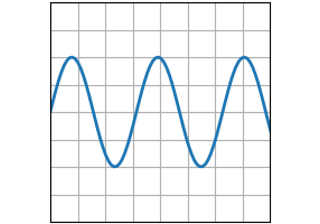plot(x, y)

plot(x, y)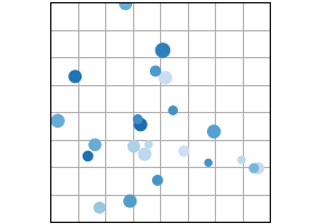scatter(x, y)

scatter(x, y)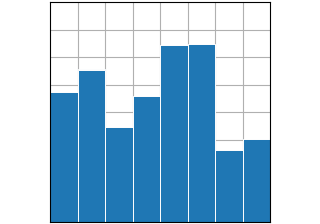bar(x, height)

bar(x, height)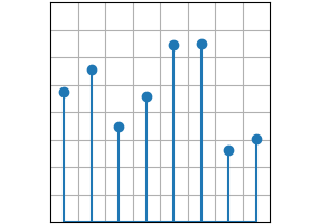stem(x, y)

stem(x, y)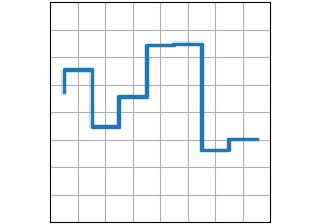step(x, y)

step(x, y)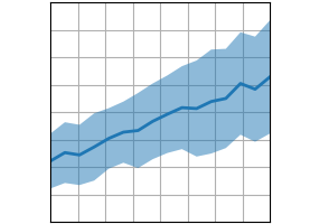fill_between(x, y1, y2)

fill_between(x, y1, y2)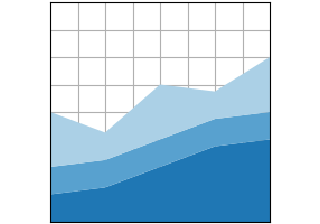stackplot(x, y)

stackplot(x, y)